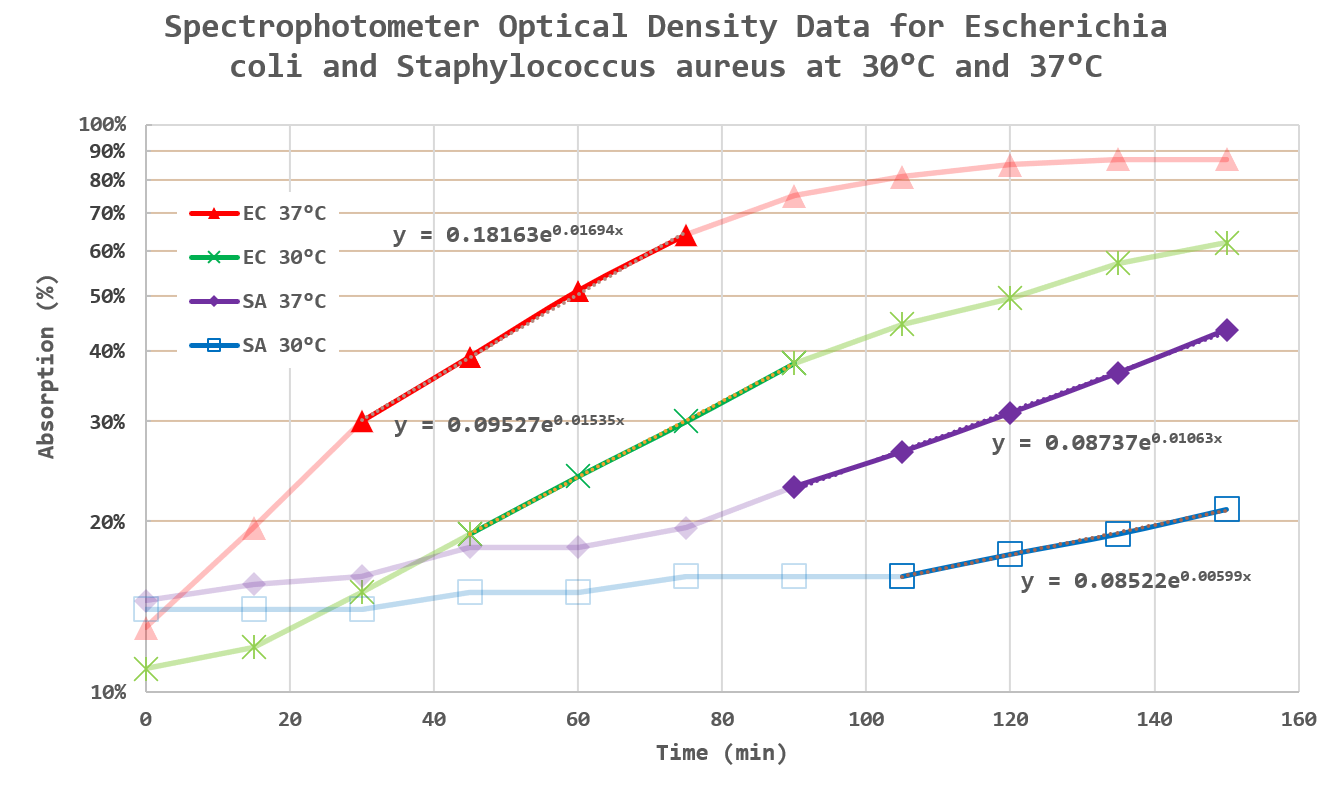# Computing Bacteria Reproduction Rate and Doubling Time

Quote of the Day

A people that elect corrupt politicians, imposters, thieves and traitors are not victims... but accomplices.

## Introduction

Until the arrival of the coronavirus, I looked forward every week to volunteering at a local library as a tutor for university students. Now that COVID is raging around me, I have moved the tutoring online. Most of the students are training for some form of a medical career. This week a student presented me with bacterial growth data and was wondering how to estimate the growth rate and doubling time for the bacteria using Excel. This exercise nicely illustrates the entire data analysis process (Figure 1) in a single example and I decided to post my solution here.

I include my workbook here for those who are interested in following the analysis.

## Background

### Bacterial Growth Characteristics

Figure 2 shows the logarithm of the bacteria count versus time (semi-log chart). The chart shows four growth phases:

• Lag (label A)
A period of minimal growth as the bacteria adapts to its new environment.
• Log (label B)
A period of exponential growth. On a semi-log plot, exponential growth plots as a straight line.
• Stationary (label C)
A period of no growth as the bacteria encounters growth limiting factors.
• Death (label D)
The bacteria die off from a lack of resources.

The analysis will focus on identifying the log phase and determining the slope of the line.

### Bacterial Growth Measurement

Because of the difficulty associated with counting individual bacteria, the data provided in this exercise comes from measuring the optical density of a bacteria sample  using a spectrophotometer (Figure 3). For this post, optical density is to be viewed as proportional to bacterial count. The details of estimating bacteria growth rate using optical density are a bit outside the scope of this blog post. For more information, see this website.

### Bacterial Growth Data

Figure 4 shows the optical density data for samples of two types of bacteria (EC = Escherichia coli, SA = Staphylococcus aureus) at two different temperatures (30°C and 37°C).

## Analysis

### Exploratory Data Analysis/Modeling

The student told me that she could only present one graph, so that graph had to perform multiple functions. Figure 5 shows my approach, which consists of:

• Plotting every data point, with the log phase points darker than the rest.
The region of linear growth is estimated by eyeball.
• Fit an exponential function to the log phase points using Excel's trendline feature.
An exponential function graphs as a line on a semilog plot. This line is shown as a thick bar. The equation of the line has the form $latex N=A\cdot {{e}^{{m\cdot t}}}$, where N = bacteria count, A = constant, m = exponential growth term, and t = time.
• Each plot on the graph is labeled with its corresponding least-square fit exponential equation.Figure 4: Graph of Data, Identification of Log Phase, and Exponential Curve Fits.

### Doubling Time Estimate

The course assignment also wanted the bacteria doubling time (τ) calculated. Equation 1 shows the derivation of the relationship between doubling time and the exponential growth term (m).

 Eq. 1 $latex \displaystyle N=A\cdot {{e}^{{m\cdot t}}}&s=2$ $latex \displaystyle 2\cdot N=A\cdot {{e}^{{m\cdot \left( {t+\tau} \right)}}}&s=2$ $latex \displaystyle \frac{{2\cdot N}}{N}=\frac{{A\cdot {{e}^{{m\cdot \left( {t+\tau} \right)}}}}}{{A\cdot {{e}^{{m\cdot t}}}}}&s=2$ $latex \displaystyle 2={{e}^{{m\cdot \tau }}}&s=2$ $latex \displaystyle \therefore \tau =\frac{{\ln \left( 2 \right)}}{m}&s=2$

Using Equation 1, we can compute the doubling times for the four test cases shown above.

## Conclusion

This bacterial growth problem provided a good example of how to apply Excel to a simple laboratory data analysis problem.

This entry was posted in Excel, General Mathematics, Health, optics, Statistics. Bookmark the permalink.

### 2 Responses to Computing Bacteria Reproduction Rate and Doubling Time

1.Norrie Steed says:

Excellent article . I remember doing this during my biology degree( was mainly microbiology .... and over 40 years ago now 😂)

•mathscinotes says:

I really enjoy working on these problems with the students. This particular student just wrote to me that she was accepted into a nursing graduate school and wanted to say thanks for all the help. She immigrated to the US from Kenya with nothing and is now well on her way to a career in nursing.

mark